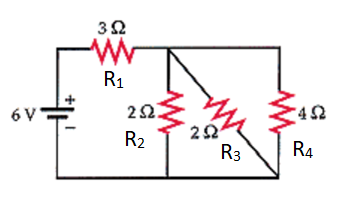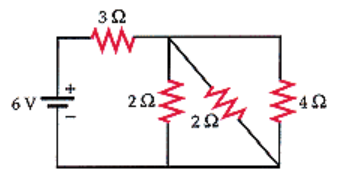# Problem: The battery in the figure below has negligible internal resistance.Find the current in each resistor.  1)A (3 Ω resistor)2)A (4 Ω resistor)3)A (vertical 2 Ω resistor)4) A (diagonal 2 Ω resistor)

###### FREE Expert Solution

Equivalent resistance for resistors in parallel:

$\overline{)\frac{\mathbf{1}}{{\mathbf{R}}_{\mathbf{eq}}}{\mathbf{=}}\frac{\mathbf{1}}{{\mathbf{R}}_{\mathbf{1}}}{\mathbf{+}}\frac{\mathbf{1}}{{\mathbf{R}}_{\mathbf{2}}}{\mathbf{+}}{\mathbf{.}}{\mathbf{.}}{\mathbf{.}}{\mathbf{+}}\frac{\mathbf{1}}{{\mathbf{R}}_{\mathbf{n}}}}$

Equivalent resistance for resistors in series:

$\overline{){{\mathbf{R}}}_{{\mathbf{eq}}}{\mathbf{=}}{{\mathbf{R}}}_{{\mathbf{1}}}{\mathbf{+}}{{\mathbf{R}}}_{{\mathbf{2}}}{\mathbf{+}}{\mathbf{.}}{\mathbf{.}}{\mathbf{.}}{\mathbf{+}}{{\mathbf{R}}}_{{\mathbf{n}}}}$1)

R2, R3 and R4 are in parallel:

R234 = (1/R2 + 1/R3 + 1/R4)-1 = (1/2 + 1/2 + 1/4) = 0.8Ω

R1 and R234 are in series:

87% (411 ratings)###### Problem Details

The battery in the figure below has negligible internal resistance.Find the current in each resistor.

1)A (3 Ω resistor)

2)A (4 Ω resistor)

3)A (vertical 2 Ω resistor)

4) A (diagonal 2 Ω resistor)